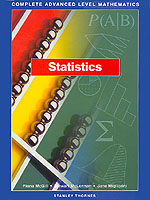Statistics

By Fiona McGill, Stewart McLennan & Jane Migliorini
July 1999
ISBN: 0-7487-3560-7552 p. illus.
\$63.50 Paper Original

A comprehensive textbook on statistics suitable for high school or college courses. Contents include: Principles of Statistics; Collection of Data; Data Analysis I; Data Analysis II; Probability; Permutations and Combinations; Discrete Probability Distributions; Binomial, Geometric and Poisson Distributions; Continuous Probability Distributions; The Normal Distribution; Correlation and Regression; Estimation I: Point Estimators; Estimation II: Interval Estimation; Significance Tests I: Single Values; Significance Tests II: Two Values; Chi-Squared Test and Analysis of Errors; Answers; Formulas; Tables.